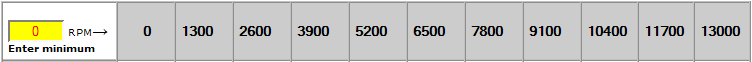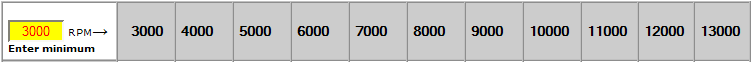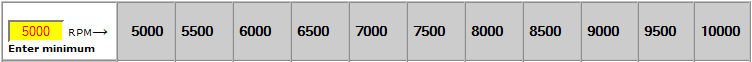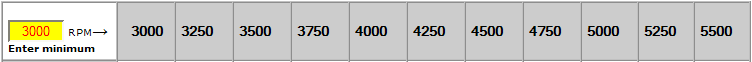Resulting speeds: Calculate resulting speeds at adjustable RPM in all 3 setups Default, table "Speed in Mph at RPM in Gear in selected setup:" will show your speeds in all gears at several default RPM's between zero RPM and M.P.RPM. This may result in a strange 'pitch' between the RPM values. Let's say your bike does 13000 M.P. RPM. This will result in the following table header:The RPM range is always divided by 10. The RPM range = M.P.RPM - Min RPM = 13000 - 0 = 13000. This divided by 10 give a pitch of 13000 /10 = 1300 RPM. Now say you want to read your speeds at exactly 3000,4000, 5000, 6000, 7000, 8000 and so on RPM. To do so change the default minimum RPM manually to say 3000. This will result in the following header and according speeds:In this case the RPM range = 13000 - 3000 = 10000. This is divided by 10 so you get a header with nice round numbers. Now if you want to be more accurate, say every 500 RPM in the range of 5000 - 10000 RPM, you would change Minimum RPM to 5000 and the Maximum to 10000. This does not mean your bike will be slower, it just limits the display in the table to this range !For the remaining RPM range you could enter 8000 and 13000 as min. and M.P.RPM. The way to find minimum and M.P. RPM to enter to see the speed results at the RPM's you want to, is: First decide on the "RPM pitch", say every 1000 RPM, every 500 RPM or every 250 RPM and so on, Decide on the start of the RPM range you want view, this is the value for Minimum RPM Calculate M.P.RPM = Minimum RPM + (10 * RPM Pitch) Example:    You want to see speeds at every 250 RPM in a range starting from 3000 RPM: RPM Pitch = 250 Minimum RPM = 3000 M.P.RPM = 3000 + (10 * 250) = 5500. This will result in:To see the remaining RPM range, you could enter Minimum = 5500 and M.P.RPM = 5500 + (10*250) = 8000.